# Charting

## Plotly

### Introduction

plotly is an online charting tool with a python API. It offers the ability to create rich and interactive graphs.

### Import Libraries

Import the plotly library.

import plotly.express as px
import plotly.graph_objects as go

### Get Historical Data

Get some historical market data to produce the plots. For example, to get data for a bank sector ETF and some banking companies over 2021, run:

qb = QuantBook()
tickers = ["XLF",   # Financial Select Sector SPDR Fund
"COF",   # Capital One Financial Corporation
"GS",    # Goldman Sachs Group, Inc.
"JPM",   # J P Morgan Chase & Co
"WFC"]   # Wells Fargo & Company
symbols = [qb.AddEquity(ticker, Resolution.Daily).Symbol for ticker in tickers]
history = qb.History(symbols, datetime(2021, 1, 1), datetime(2022, 1, 1))

### Create Candlestick Chart

You must import the plotting libraries and get some historical data to create candlestick charts.

In this example, you create a candlestick chart that shows the open, high, low, and close prices of one of the banking securities. Follow these steps to create the candlestick chart:

1. Select a Symbol.
2. symbol = symbols
3. Slice the history DataFrame with the symbol.
4. data = history.loc[symbol]
5. Call the Candlestick constructor with the time and open, high, low, and close price Series.
6. candlestick = go.Candlestick(x=data.index,
open=data['open'],
high=data['high'],
low=data['low'],
close=data['close'])
7. Call the Layout constructor with a title and axes labels.
8. layout = go.Layout(title=go.layout.Title(text=f'{symbol.Value} OHLC'),
xaxis_title='Date',
yaxis_title='Price',
xaxis_rangeslider_visible=False)
9. Call the Figure constructor with the candlestick and layout.
10. fig = go.Figure(data=[candlestick], layout=layout)
11. Call the show method.
12. fig.show()

The Jupyter Notebook displays the candlestick chart.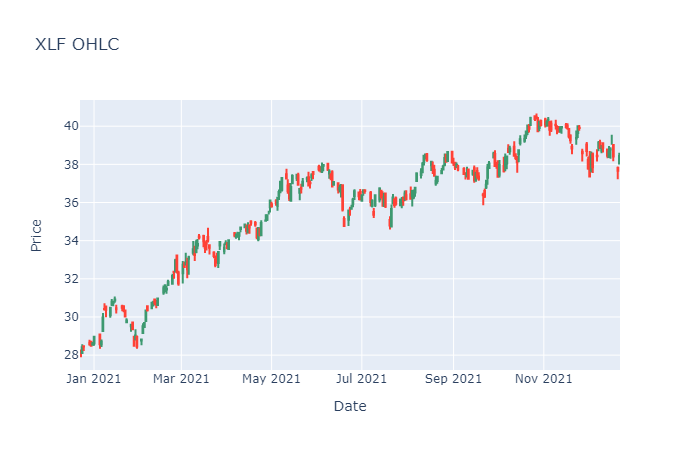### Create Line Chart

You must import the plotting libraries and get some historical data to create line charts.

In this example, you create a line chart that shows the closing price for one of the banking securities. Follow these steps to create the line chart:

1. Select a Symbol.
2. symbol = symbols
3. Slice the history DataFrame with the symbol and then select the close column.
4. data = history.loc[symbol]['close']
5. Call the DataFrame constructor with the data Series and then call the reset_index method.
6. data = pd.DataFrame(data).reset_index()
7. Call the line method with data, the column names of the x- and y-axis in data, and the plot title.
8. fig = px.line(data, x='time', y='close', title=f'{symbol} Close price')
9. Call the show method.
10. fig.show()

The Jupyter Notebook displays the line chart.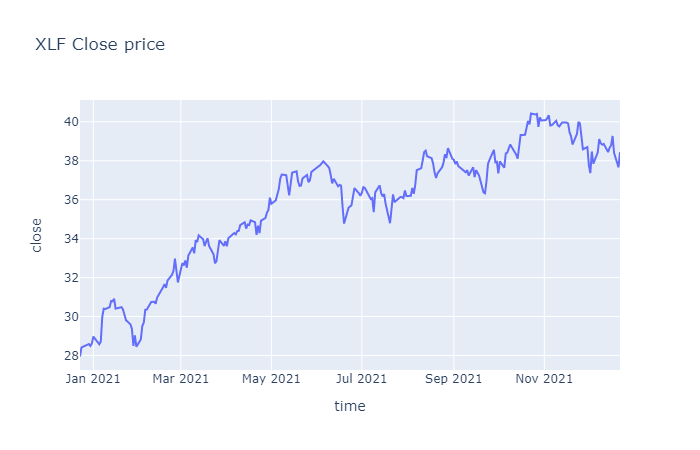### Create Scatter Plot

You must import the plotting libraries and get some historical data to create scatter plots.

In this example, you create a scatter plot that shows the relationship between the daily returns of two banking securities. Follow these steps to create the scatter plot:

1. Select 2 Symbols.
2. For example, to select the Symbols of the first 2 bank stocks, run:

symbol1 = symbols
symbol2 = symbols
3. Slice the history DataFrame with each Symbol and then select the close column.
4. close_price1 = history.loc[symbol1]['close']
close_price2 = history.loc[symbol2]['close']
5. Call the pct_change and dropna methods on each Series.
6. daily_return1 = close_price1.pct_change().dropna()
daily_return2 = close_price2.pct_change().dropna()
7. Call the scatter method with the 2 return Series, the trendline option, and axes labels.
8. fig = px.scatter(x=daily_return1, y=daily_return2, trendline='ols',
labels={'x': symbol1.Value, 'y': symbol2.Value}) 
9. Call the update_layout method with a title.
10. fig.update_layout(title=f'{symbol1.Value} vs {symbol2.Value} Daily % Returns');
11. Call the show method.
12. fig.show()

The Jupyter Notebook displays the scatter plot.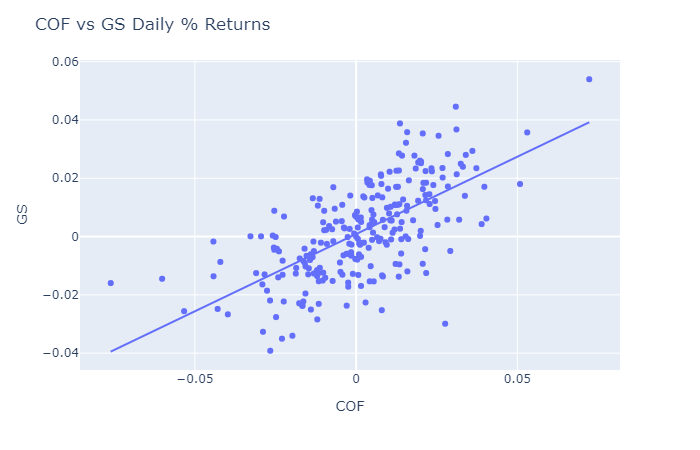### Create Histogram

You must import the plotting libraries and get some historical data to create histograms.

In this example, you create a histogram that shows the distribution of the daily percent returns of the bank sector ETF. Follow these steps to create the histogram:

1. Select the Symbol.
2. symbol = symbols
3. Slice the history DataFrame with the symbol and then select the close column.
4. data = history.loc[symbol]['close']
5. Call the pct_change method and then call the dropna method.
6. daily_returns = data.pct_change().dropna()
7. Call the DataFrame constructor with the data Series and then call the reset_index method.
8. daily_returns = pd.DataFrame(daily_returns).reset_index()
9. Call the histogram method with the daily_returns DataFrame, the x-axis label, a title, and the number of bins.
10. fig = px.histogram(daily_returns, x='close',
title=f'{symbol} Daily Return of Close Price Distribution',
nbins=20)
11. Call the show method.
12. fig.show()

The Jupyter Notebook displays the histogram.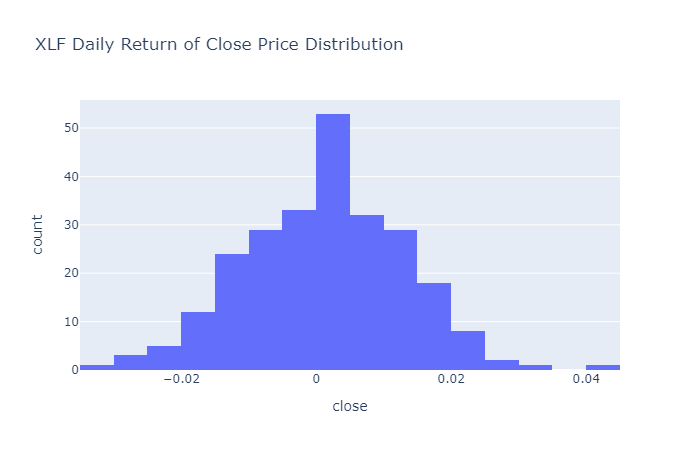### Create Bar Chart

You must import the plotting libraries and get some historical data to create bar charts.

In this example, you create a bar chart that shows the average daily percent return of the banking securities. Follow these steps to create the bar chart:

1. Select the close column and then call the unstack method.
2. close_prices = history['close'].unstack(level=0)
3. Call the pct_change method and then multiply by 100.
4. daily_returns = close_prices.pct_change() * 100
5. Call the mean method.
6. avg_daily_returns = daily_returns.mean()
7. Call the DataFrame constructor with the avg_daily_returns Series and then call the reset_index method.
8. avg_daily_returns = pd.DataFrame(avg_daily_returns, columns=["avg_daily_ret"]).reset_index()
9. Call the bar method with the avg_daily_returns and the axes column names.
10. fig = px.bar(avg_daily_returns, x='symbol', y='avg_daily_ret')
11. Call the update_layout method with a title.
12. fig.update_layout(title='Banking Stocks Average Daily % Returns');
13. Call the show method.
14. fig.show()

The Jupyter Notebook displays the bar plot.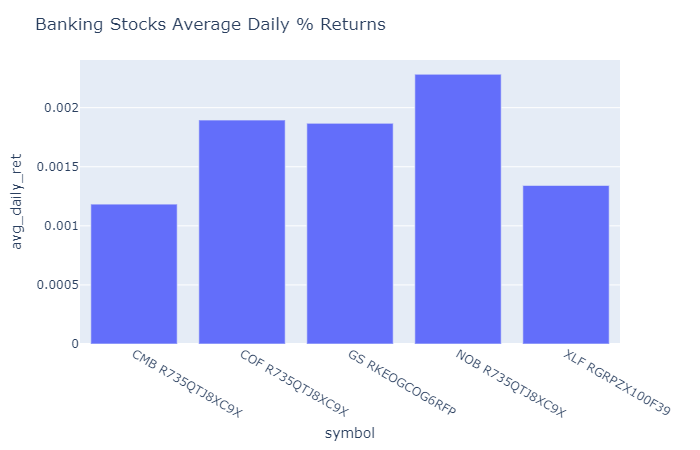### Create Heat Map

You must import the plotting libraries and get some historical data to create heat maps.

In this example, you create a heat map that shows the correlation between the daily returns of the banking securities. Follow these steps to create the heat map:

1. Select the close column and then call the unstack method.
2. close_prices = history['close'].unstack(level=0)
3. Call the pct_change method.
4. daily_returns = close_prices.pct_change()
5. Call the corr method.
6. corr_matrix = daily_returns.corr()
7. Call the imshow method with the corr_matrix and the axes labels.
8. fig = px.imshow(corr_matrix, x=tickers, y=tickers)
9. Call the update_layout method with a title.
10. fig.update_layout(title='Banking Stocks and bank sector ETF Correlation Heat Map');
11. Call the show method.
12. fig.show()

The Jupyter Notebook displays the heat map.### Create Pie Chart

You must import the plotting libraries and get some historical data to create pie charts.

In this example, you create a pie chart that shows the weights of the banking securities in a portfolio if you allocate to them based on their inverse volatility. Follow these steps to create the pie chart:

1. Select the close column and then call the unstack method.
2. close_prices = history['close'].unstack(level=0)
3. Call the pct_change method.
4. daily_returns = close_prices.pct_change()
5. Call the var method and then take the inverse.
6. inverse_variance = 1 / daily_returns.var()
7. Call the DataFrame constructor with the inverse_variance Series and then call the reset_index method.
8. inverse_variance = pd.DataFrame(inverse_variance, columns=["inverse variance"]).reset_index()
9. Call the pie method with the inverse_variance DataFrame, the column name of the values, and the column name of the names.
10. fig = px.pie(inverse_variance, values='inverse variance', names='symbol')
11. Call the update_layout method with a title.
12. fig.update_layout(title='Asset Allocation of bank stocks and bank sector ETF');
13. Call the show method.
14. fig.show()

The Jupyter Notebook displays the pie chart.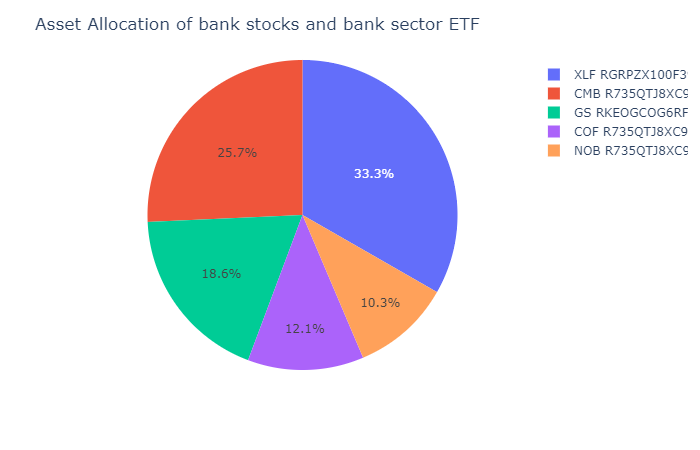You can also see our Videos. You can also get in touch with us via Discord.